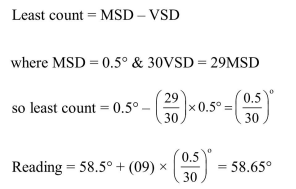# A spectrometer gives the following reading

Question:

A spectrometer gives the following reading when used to measure the angle of a prism. Main scale reading : $58.5$ degree Vernier scale reading : 09 divisions Given that 1 division on main scale corresponds to $0.5$ degree. Total divisions on the vernier scale is 30 and match with 29 divisions of the main scale. The angle of the prism from the above data :

1. 59 degree

2. $58.59$ degree

3. $58.77$ degree

4. $58.65$ degree

Correct Option: , 4

Solution: